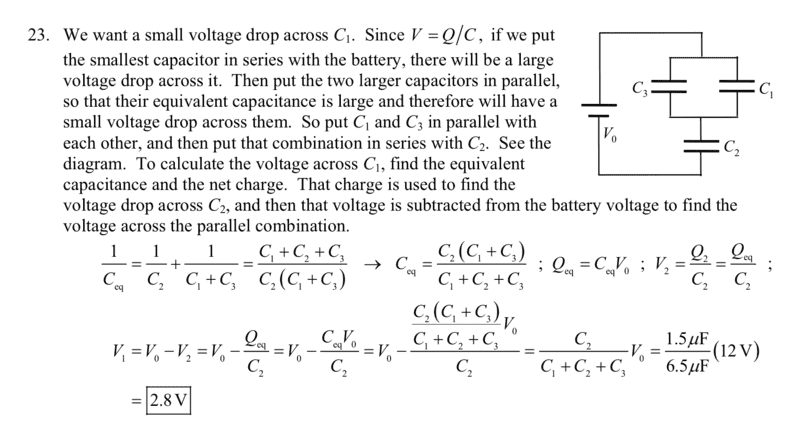# Minimizing the voltage drop across a capacitor (solution shown)

• Sunwoo Bae

#### Sunwoo Bae

Homework Statement
Shown in the text
Relevant Equations
Q = CV

capacitors in series
capacitors in parallel
The following is the question and the solution to the question.I understand the solution to the part where you find the Ceq and derive Qeq from the equation Q = Ceq*V.
However, I do not understand where V1 = V0-V2 come from.
When calculating the minimum voltage, how do you come up with the equation V1 = V0-V2, and why is V3 not taken to account?

I do not understand where V1 = V0-V2 come from.
given and total potential difference is always same.
Here given potential difference is ##V_0## and total potential difference is ##V_1+V_2##

So ##V_0=V_1+V_2##

Homework Statement:: Shown in the text
Relevant Equations:: Q = CV

capacitors in series
capacitors in parallel

The following is the question and the solution to the question.
View attachment 296217

I understand the solution to the part where you find the Ceq and derive Qeq from the equation Q = Ceq*V.
However, I do not understand where V1 = V0-V2 come from.
When calculating the minimum voltage, how do you come up with the equation V1 = V0-V2, and why is V3 not taken to account?
Are you familiar with Kirchhoff’s laws?